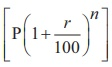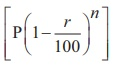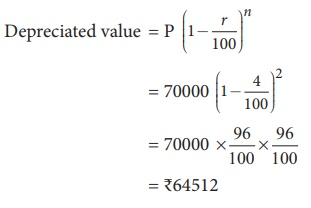Home | | Maths 8th Std | Applications of Compound Interest Formula

# Applications of Compound Interest Formula

The compound interest formula is used in the following situations.

Applications of Compound Interest Formula

The compound interest formula is used in the following situations.

(i) To find the increaseor decreasein population.

(ii) To find the growth of cells when the rate of growth is given.

(iii) To find the depreciation in the values of machines, vehicles, utility appliances etc.,

Example 4.15

The value of a motor cycle 2 years ago was ₹70000. It depreciates at the rate of 4% p.a.Find its present value.

Solution:= ₹645121

Example 4.16

The bacteria in a culture grows by 5% in the first hour, decreases by 8% in the second hour and again increases by 10% in the third hour. Find the count of the bacteria at the end of 3 hours, if its initial count was 10000.

Solution:

Bacteria at the end of 3 hoursA = ₹10626

Example 4.17

The population of a town is increasing at the rate of 6% p.a. It was 238765 in the year 2018. Find the population in the year 2016 and 2020.

Solution:

Let the population in 2016 be P.= 238765 × 53/50 × 53/50

= 95.506 × 53 × 53

A = 268276

The population in the year 2016 was 212500 and that in the year 2020 will be 268276.

Tags : Questions with Answers, Solution | Life Mathematics | Chapter 4 | 8th Maths , 8th Maths : Chapter 4 : Life Mathematics
Study Material, Lecturing Notes, Assignment, Reference, Wiki description explanation, brief detail
8th Maths : Chapter 4 : Life Mathematics : Applications of Compound Interest Formula | Questions with Answers, Solution | Life Mathematics | Chapter 4 | 8th Maths